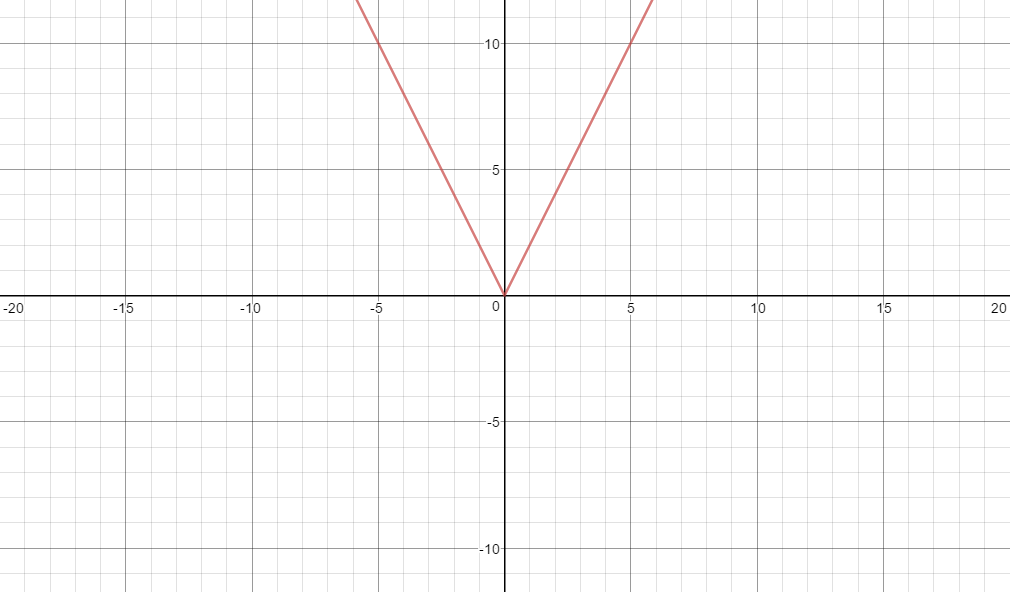# What happens to an absolute value graph if $|x|$ has a coefficient

I skipped Algebra I in school, and we have Mathematics midterms next week. While going through our review packet, I noticed graphing absolute values, something I had never seen before.

I've figured out the basics: $|x+n|$ translates the graph $n$ units along the x axis, $|x|+d$ translates the graph $d$ units along the y axis, and $-|x|$ flips the graph so it opens downward.

What happens, however, if we have $a|x|$, or $|ax|$? Is there an easy short hand way to draw this, or do I have to make a chart of the points and graph them one by one?

• If $a$ is large, then the lines of the graph have large slope ($=a$). If $a$ is small, the lines don't have large slope. $a|x|$ will decrease (how rapidly depends on $a$) to zero , where it takes the value zero, then increase with the same speed in the positive direction. $|ax|= |a||x|$, so it is the same. If $a$ is negative, then assume $a$ is positive, draw the graph and then flip it. Jan 20, 2017 at 1:15
• What happens to the graph of the line $y=x$ if $x$ has a coefficient different from $1$?
– amd
Jan 20, 2017 at 1:35
• +1 Bice observations. These changes to the graph work for all functions $f(x)$, not just for the absolute value. Jan 20, 2017 at 1:44

$|x+n|$ translates the graph $n$ units along the x axis, $|x|+d$ translates the graph $d$ units along the y axis, and $-|x|$ flips the graph so it opens downward.

$\lvert x + n \rvert$ is the graph of $\lvert x \rvert$ translated $n$ units to the left if $n > 0$. If $n< 0$ it will translate to the right. $n=0$ changes nothing. E.g. the tip will move from $x=0$ to $x=-n$.You can fiddle with it here.

$\lvert x \rvert + d$ is the graph of $\lvert x \rvert$ translated $d$ units upwards if $d > 0$. If $d < 0$ it will get translated downwards. E.g. the the tip will move from $y=0$ to $y = d$.You can fiddle with it here.

$-\lvert x \rvert$ is the graph of $\lvert x \rvert$ mirrored along the $x$-axis.What happens, however, if we have $a|x|$, or $|ax|$?

$a\lvert x \rvert$ squeezes the graph of $\lvert x \rvert$ horizontally with growing $a$, if $a>1$. E.g. $(1,1)$ will get mapped to $(1,a)$. If $a = 1$ nothing changes. If $a \in (0,1)$ then the graph will widen horizontally. If $a = 0$ the graph will flatline to the constant zero function.

If $a$ is negative one will have an additional mirroring at the $x$-axis.You can fiddle with it here.

$\lvert a x \rvert$ is just $\lvert a\rvert \lvert x \rvert$. This leads to a different dynamics, as there are no negative factors anymore compared to the previous example.

You can fiddle with it here.

• this got good. :)
– SAJW
Jan 20, 2017 at 1:42
• Yup. We good now! :D Jan 20, 2017 at 1:46

I just realized this, moments after posting.

If $a$ exists in the context of the question, it affects the graph as $m$ would in a linear equation.

In $a = 1$ or $a = -1$, the slope on either side is $1$ or $-1$ respectively. The value of $a$ represents the slope on the right hand side of the line of symmetry and the opposite of the slope on the left hand side. (For $y=|ax|$, we can assume $y=|a||x|$, and simply find the abolsute value of $a$, and get $y=a|x|$)

For example, if $a=1$:But, if $a=2$,• :-P Well, you got that right. Jan 20, 2017 at 1:20

For starters,

$$|ax|=|a||x|=\begin{cases}+|a|x;&x\ge0\\-|a|x;&x<0\end{cases}\implies\text{slope is }\pm a$$

which is just a taller or shorter $V$ shaped graph.

• Lmao, when you look at your own post and you think "Oh! That one looks good!" and you then try to upvote, only to startling surprise that you posted this answer. Jan 20, 2017 at 1:45